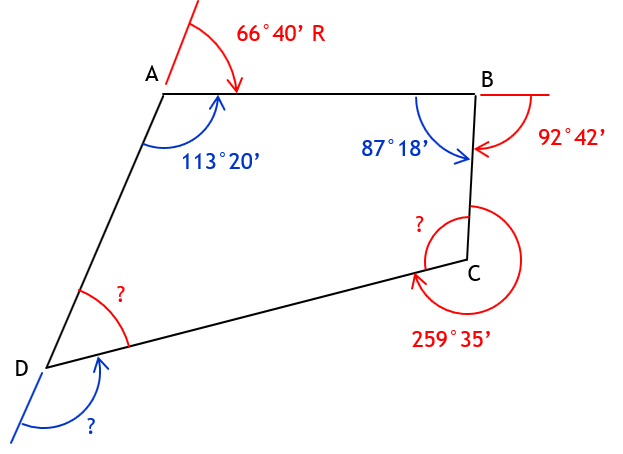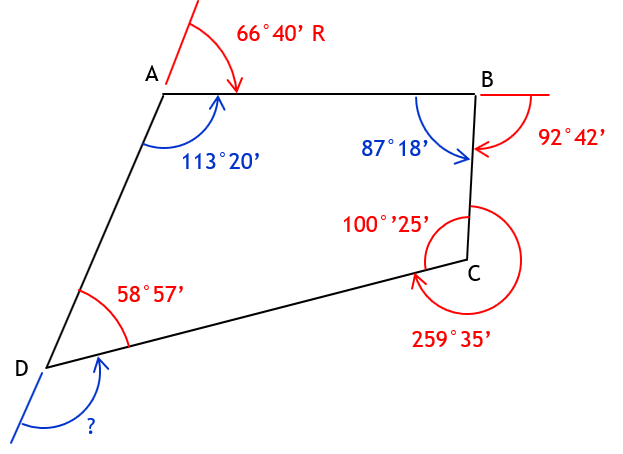## 3. Example Problem

Given this polygon with combination of angle types:Determine

#### a. Deflection angle at A from D to BDefl angle A = 180°00’ - 113°20’ = 66°40’ = 66°40’ R

#### b. Angle left at B from A to CInt angle B = 180°00’ - 92°42’ = 87°18’

#### c. Deflection angle at D from A to CNeed interior angle at D to compute the deflection angle.To compute interior angle at D, subtract the sum of the other interior angles from (n-2)x180°.

Need interior angle at C.Int angle C = 360°00’ - 259°35’ = 100°25’Int angle D = (4-2)x180°00’ - (113°20’ + 87°18’ + 100°25’) = 360°00’ - 301°03’ = 58°57’Defl angle D = 180°00’ - 58°57 = 121°03’ = 120°03’ L